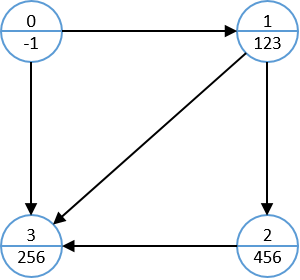### 题目大意

Q老师编写的软件中存在着N个模块，依次编号为1..N，其中编号为i的模块会在接收到一个名称为Signal[i]的信号时启动，对于不同的模块，他们启动需要的信号可能是相同的。 在这N个模块里，代码的执行也会触发若干(K<=5)信号，这些信号又可能会导致其他模块的启动，从而使得整个软件运转起来，但值得庆幸的是，模块间的互相启动是不存在循环的，也就是无论收到什么样的信号，这个软件最终都会停止运行。 现在Q老师为了测试整个软件，使用特定的工具发出了M个信号，而他希望能够知道，每个模块应该被运行的次数。

### 解题思路

• 初始信号流，即 M 个初始信号，我们将其设定为模块`0`发出的信号
• 若模块`i`发出的信号能够使得模块`j`被激活，我们连接一条从`i``j`的有向边`(i,j)`

``````3 2
123 256
123 2 456 256
456 3 666 111 256
256 1 90
````````````Input n
Input m
// 将初始数据流做为 module 0
For i = 1 .. m
Input sign
module.sendSign.push(sign)
End For
// 读取其他module的信息
For i = 1 .. n
Input sign
module[i].activeSign = sign
signList[ sign ].canActiveModule.push(i)
Input num
For j = 1 .. num
Input sign
module[i]sendSign.push(sign)
End For
End For
// 构造有向无环图
For i = 0 .. n
For j in signList[ sign ].canActiveModule
End For
End For
End For
``````

``````DFS(nowModule):
If (nowModule not 0) Then
activeCount[ nowModule ] = activeCount[ nowModule ] + 1
End If
For each j in (nowModule, j)
DFS(j)
End For
``````

``````// 在构造图时同时统计入度
For i = 0 .. n
For j in signList[ sign ].canActiveModule
inDegree[j] = inDegree[j] + 1
End For
End For
End For

// 进行拓扑排序
tail = 0;
For i = 0 .. n  // 这里一定要从0开始，因为Module 0也是图中的点
If (inDegree[i] == 0) Then  // 入度为0的点
sequence[tail] = i
tail = tail + 1
End If
End For

activeCount = 1  // 设定初始信号流的访问次数为1
activeCount[1 .. n] = 0
i = 0
While (i < tail) Then
nowModule = sequence[i]
For each j in (nowModule, j)
activeCount[j] = activeCount[j] + activeCount[ nowModule ]
inDegree[j] = inDegree[j] - 1
If (inDegree[j] == 0) Then
sequence[tail] = j
tail = tail + 1
End If
End For
i = i + 1
End While
``````

@lucienwang 我还是贴代码吧，帮忙找找问题出在哪。。。多谢！

``````#include<stdio.h>
#include<stdlib.h>

/*
* Record signal message in linktable. This is the defination
* of linktable node. The input signal is the index of array: signals.
* Parameters:
*      @input: The number of signals that can call this singal.
*      @value: The value of this signal.
*      @next: The pointer of the output of this signal.
*      @count: The number of calling of this signal.
*/
typedef struct node1 {
int input;
int value;
struct node1* next;
int count;
} signal;

// The signals list.
signal signals;

// The module list, the element is the input signal of each module.
int modules;

// The number of modules.
int N;

/*
* This fuction deals with one test case.
* Parameters:
*      None.
* Return:
*      None.
*/
void function(void);

/*
* This function initial the situation for one test case.
* Parameters:
*      None.
* Return:
*      None.
*/
void init(void);

/*
* This function adds one output signal of a signal.
* Parameters:
*      @input: The input signal.
*      @output: The output signal number to add.
*/
void add_output_signal(int input, int output);

/*
* This function calculates the number of each module calling.
* Parameters:
*      @input: The input signal.
* Return:
*      None.
*/
void calculate(int input);

/*
* This function print the result of one test case.
* Parameters:
*      None.
* Return:
*      None.
*/
void print_result(void);

/*
* The main program.
*/
int main(void) {
int T;
scanf("%d", &T);

for(int i = 0; i < T; i++) {
function();
}

return 0;
}

/*
* This fuction deals with one test case.
* Parameters:
*      None.
* Return:
*      None.
*/
void function(void) {
init();
int M;
scanf("%d %d", &N, &M);

signals.input = 0;
signals.count = 1;
int s;
for(int i = 0; i < M; i++) {
scanf("%d", &s);
}

int S, K, E;
for(int i = 0; i < N; i++) {
scanf("%d %d", &S, &K);
modules[i] = S;
for(int j = 0; j < K; j++) {
scanf("%d", &E);
}
}

int tag = 1;
while(tag == 1) {
tag = 0;
for(int i = 0; i < 100005; i++) {
if(signals[i].input == 0) {
tag = 1;
calculate(i);
}
}
}
print_result();
}

/*
* This function initial the situation for one test case.
* Parameters:
*      None.
* Return:
*      None.
*/
void init(void) {
for(int i = 0; i < 100005; i++) {
signals[i].input = -1;
signals[i].value = i;
signals[i].count = 0;
signals[i].next = NULL;
modules[i] = 0;
}
N = 0;
}

/*
* This function adds one output signal of a signal.
* Parameters:
*      @input: The input signal.
*      @output: The output signal number to add.
*/
void add_output_signal(int input, int output) {
signal* s = (signal*) malloc(sizeof(signal));
s->value = output;

s->next = signals[input].next;
signals[input].next = s;

signals[output].input++;
if(signals[output].input == 0) {
signals[output].input++;
}
}

/*
* This function calculates the number of each module calling.
* Parameters:
*      @input: The input signal.
* Return:
*      None.
*/
void calculate(int input) {
signal* p = signals[input].next;
while(p != NULL) {
signals[p->value].input--;
signals[p->value].count += signals[input].count;
if(signals[p->value].count >= 142857) {
signals[p->value].count %= 142857;
}
p = p->next;
}
signals[input].input = -1;
}

/*
* This function print the result of one test case.
* Parameters:
*      None.
* Return:
*      None.
*/
void print_result(void) {
for(int i = 0; i < N; i++) {
printf("%d ", signals[modules[i]].count);
}
printf("\n");
}
``````